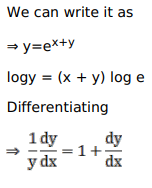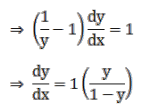# Solve this following

Question:

Mark $(\sqrt{)}$ against the correct answer in the following:

If $y=e^{x}+e^{x+\ldots \infty}$ then $\frac{d y}{d x}=?$

A. $\frac{1}{(1-y)}$

B. $\frac{y}{(1-y)}$

C. $\frac{y}{(y-1)}$

D. none of these

Solution: